Published: 07 October 2016

# Experimental study of ultrasonic relaxation of residual stresses in the elastic plates

Albert Korolev1
Andrei Balaev2
Timur Baltaev3
Boris Iznairov4
1, 2, 3, 4Yuri Gagarin State Technical University of Saratov, Saratov, Russia
Corresponding Author:
Andrei Balaev
Views 28

#### Abstract

The article describes a method of ultrasonic relaxation of residual stresses in elastic circular plates used in the sensors of gas and liquid overpressure control. The method is based on the use of the energy of ultrasonic vibrations imparted to an elastic plate, which causes gradual accumulation and subsequent relaxation of residual stresses and strains. In order to test the adequacy of the mathematical description of the ultrasonic relaxation mechanism, and to establish the dependencies of residual strain on technological factors, an experiment has been carried out. An ultrasonic setup was developed to perform the experimental part. As a result of the experiment an empirical power-law dependence of residual strain on the amplitude of the ultrasonic vibrations, the duration of machining and the static load was obtained. This dependency is shown on the corresponding graphs. The graphs demonstrate the behavior of the residual strains at different technological modes of ultrasonic processing.

## 1. Introduction

Elastic sensing elements such as circular plates are among the most critical elements of the pressure sensors used in test equipment. The accuracy of test indications depends on the quality of the elastic elements, so to ensure the stability of their mechanical properties throughout the entire period of operation the requirements should be increased. In operation, elastic elements are subjected to multiple strains, resulting in the accumulation of residual strains, which affect the accuracy of test indications during the operation of the pressure sensor. The reason for this is the occurrence of plate deformation under inner residual stresses caused by the plastic creep of the material. To avoid this in operation, circular plates are subjected to stabilization, which is a kind of “training” when a uniformly distributed cyclic load is applied on the circular plate under the action of liquid or gas simulating normal operation of the device. As a result of this multi-cyclic loading of the elastic plate for several hours, first at negative temperature, then at elevated temperature there occurs the relaxation of residual stresses. However, this technology has low productivity and does not always ensure complete relaxation of residual stresses resulting in the reduced accuracy of test indications.

This problem can be solved using the energy of ultrasonic vibrations in order to reduce the time of multi-cyclic loading, which is confirmed by the studies [1-5]. According to the presented research results, while using the method of ultrasonic relaxation there is a significant reduction of time and energy at more stable results for the geometrical parameters of products compared to the thermal methods . However, the question remains open whether it is possible to effectively use the ultrasonic processing to stabilize the accumulated strains in parts such as circular plates, which are used as elastic sensing elements in pressure sensors.

Therefore, in order to improve the productivity and quality of residual stresses relaxation a method of ultrasonic stabilization of elastic plates has been developed . To calculate technological modes of the ultrasonic method a mathematical description of the mechanism for the ultrasonic relaxation of residual stresses in elastic plates was developed and experimental studies confirming the adequacy of the mathematical description were performed.

## 2. Experiment description

Twenty-four circular plates were selected as the object of experimental studies. These plates are used as sensing elements in sensors of gas and liquid overpressure control type MD – TP. The sensors are manufactured by the instrumentation manufacturing association “Signal” (the city of Engels), which specializes in manufacturing of control devices. The circular plates were made of steel 20X13 GOST 5632-72, hardened to a hardness of 48-50 HRC, and were subjected to all the processing steps prior to stabilization. The circular plate had the diameter $d=$29 mm and thickness $h=$0.5 mm (Fig. 1).

Fig. 1Circular plate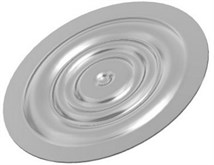The experimental part included the development and assembly of a unique setup presented in Fig. 2.

On top of the frame there is a vertical guide, along which a movable yoke with a rigid connection ultrasonic transducer moves freely. On the setup desktop a mounting plate is installed, which is attached to the frame with four bolts.

Fig. 2General view of the experimental setup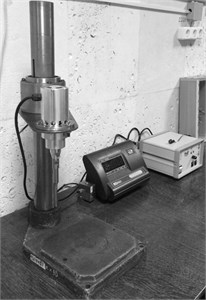A strain measuring element to control the supplied static load $P$ (N) is attached to the mounting plate. Using the adapter, the pressure sensor of the type MD – TP is mounted on the strain measuring element. At the end of the ultrasonic transducer, in the journal with bolted joints a tool in the form of a tip with a flat face made of the material VK-8 GOST 3882-74 is fixed.

When the movable yoke is moving along the vertical guide the ultrasonic transducer can be clamped to the processed surface of the circular plate. The pressing force is provided by a special load controlled in real time by the strain measuring element. The ultrasonic transducer is attached to the ultrasonic generator “MEF 15”.

To assess the influence of processing factors on the quality of relaxation of residual stresses and stabilize the geometrical parameters, the complete factorial was used. The following processing factors were selected as the most significant independent ones: a static pressing force of the tip to the plate surface $P$ (N), processing time – $t$ (s), vibration amplitude of the ultrasonic tool – $A$ (mm). The amount of residual strain of the circular plate ${\mathrm{\Delta }}_{o}$ (µm) was selected as the controlled parameter. The frequency of ultrasonic vibrations of the indenter during the whole experiment did not change and remained at 20 kHz.

The load on the plate was controlled by the strain-gauge, on which a device with the fixed plate was mounted. The vibration amplitude of the tool and the plate was measured using a special device, in which an indicating gage with a scale division of 1 µm was fixed. Residual strain after ultrasonic processing was measured by determining the distance from the center of the plate to the base surface of the mandrel, to which the plate was fixed, before and after processing.

As a result of the experiment a regression power-law dependence of the residual strain on the processing factors was obtained, the significance of its coefficients was performed on the basis of the Student’s t-test. The model was verified with the help of the F-test.

## 3. Experiment results

As a result of the experiment a regression power-law dependence of residual strain of a circular plate ${\mathrm{\Delta }}_{o}$ from three processing factors was obtained:

1
${\mathrm{\Delta }}_{o}=1.34\cdot 1{0}^{3}\cdot {t}^{0.12}\cdot {A}^{1.65}\cdot {P}^{0.34},$

where ${\mathrm{\Delta }}_{o}$ is the accumulated residual strain of the circular plate, µm; $t$ is the processing time, s; $A$ is the amplitude of the ultrasonic vibrations of the indenter, mm; $P$ is the static load on the plate, N.

The significance of the dependence coefficients Eq. (1) was performed on the basis of the Student’s t-test. The model was verified using the F-test.

Fig. 3Dependency of residual strain of the plate Δo (t, A, P): a) on the vibrations amplitude A; b) on the external load P; c) on the processing time t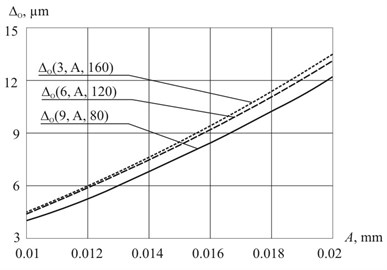a)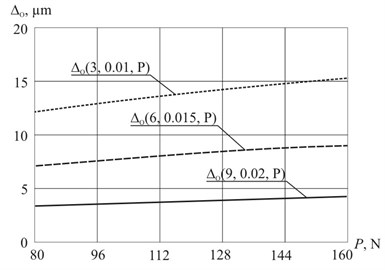b)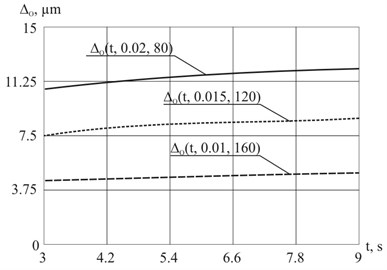c)

Fig. 3 presents the results of the experiment in graphical form. It shows the dependences of residual strain of the plate ${\mathrm{\Delta }}_{o}$ (µm) on the vibrations amplitude $A$ (mm) of the ultrasonic tool, the pressing force of the tool to the processed plate $P$ (N) and processing time $t$ (s).

The graphs show that the vibrations amplitude of ultrasonic tool substantially influences the accumulated residual strain of the plate (Fig. 3(a)). The higher the amplitude oscillations and accumulated strain, the higher the processing accuracy is. However, it requires more time to stabilize the strain of a circular plate. The main plate strain occurs in the initial time interval of processing in the range of 1-3 seconds, after which the change slows down (Fig. 3(c)). With the increase in external load, the amount of residual strain of the circular plate decreases (Fig. 3(b)) and the required processing time decreases. Therefore, this factor is critical when choosing efficient processing conditions.

Along with the empirical dependencies presented by the regression model Eq. (1) and graphs (Fig. 3), the adequacy of the developed mathematical model of the ultrasonic relaxation of residual stresses was evaluated (Fig. 4).

Fig. 4 shows, that the theoretical values of residual strain $\mathrm{\Delta }t$ ($A$) are within the confidence limits of the experimental values $\mathrm{\Delta }\lambda$ ($A$), which confirms the adequacy of the mathematical model. Based on the proven mathematical model a program for automated calculation of the parameters of stabilizing processing was developed, and the corresponding methodology was developed, which allows the calculation of the rational technological modes of ultrasonic stabilization of the circular plates. The obtained method enabled the calculation of the rational values of the ultrasonic processing modes for the considered plate: $t=$ 60 s; $A=$ 0.01 mm; $P=$ 60 N.

Fig. 4The comparison of theoretical Δt (A) and experimental Δλ (A) dependencies of residual strain of the circular plate on the vibrations amplitude A of the ultrasonic tool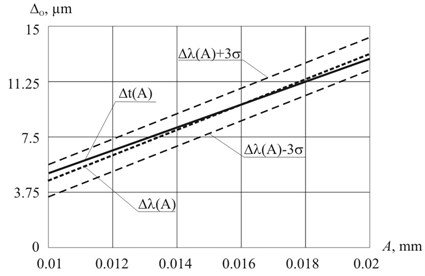## 4. Summary

Experimental research allowed establishing the relationship between the technological modes of the ultrasonic stabilization of elastic circular plates used in pressure sensors and the amount of residual strains. Based on the resulting graphs (Fig. 3) and the empirical dependency Eq. (1) the following conclusions can be drawn:

• the increase in the amplitude of the ultrasonic vibrations leads to the increase of residual strains;

• the increase of the static load leads to the increase of residual strains;

• the increase of the processing time leads to the increase of residual strains;

• the vibrations amplitude has the greatest effect on the magnitude of the residual strains in relation to the value of the static load and processing time;

• the most intensive growth of the plate strain occurs in the first 3 seconds of the ultrasonic stabilization, after which the process slows down, so that the machining time has the least impact on the magnitude of the residual strains;

• short ultrasonic processing established according to the results of the experiment confirms the high productivity and efficiency of the ultrasonic method compared to the traditional method of “training” plates;

• experimentally obtained dependency of residual strains on the process induced distortion confirms the theoretical dependency.

## 5. Conclusions

As a result of experiments a dependency of residual strains in circular elastic plates of the pressure sensors on the processing factors was established. This proves the validity of the theoretical model of ultrasonic stabilization.

31 July 2016
Accepted
03 September 2016
Published
07 October 2016
SUBJECTS
Mechanical vibrations and applications
Keywords
ultrasonic processing
residual stresses
stress relaxation
elastic strain
residual strain
Acknowledgements

This work was executed at financial support of the Ministry of Education and Science of the Russian Federation, Agreement No. 9.896.2014/K.